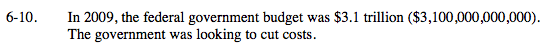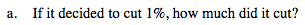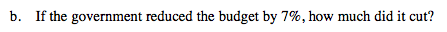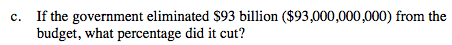### Home > MC2 > Chapter 6 > Lesson 6.1.1 > Problem6-10

6-10.Find 1% of $3.1 trillion. Convert 1% into a decimal, then multiply that by$3.1 trillion.

(0.01)(3,100,000,000,000)

31 billion or 31,000,000,000.Find 7% of $3.1 trillion. See part (a) for help.$93 billion is what percent of $3.1 trillion? Divide$93 billion by \$3.1 trillion.

$\frac{93,000,000,000}{3,100,000,000,000}=0.03$

Convert this decimal into a percentage.